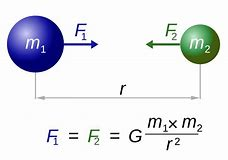# Universal Law of Gravitation | Newton’s Law of Gravitation

## Gravitation

Sir Isaac Newton’s Universal Law of Gravitation can be applied both at cosmic level and atomic level. It follows inverse square law of force.

In daily life we observe things falling from a height. We often drop a pen or a pencil. A ball thrown up comes down. Isaac Newton saw an apple falling from a tree. He thought why not the apples go up?

The invisible force that attracts an apple towards the earth led to the idea of gravitation.

Gravitation is the force of attraction between any two objects. It exists between an apple and the earth as well as the moon and the earth.

In the solar system, the planets go around the sun due to gravitation. In general, every object in the universe attracts every other object with a force.

Sir Isaac Newton formulated a universal law of gravitation also called Newton’s Law of Gravitation.

## Universal Law of Gravitation

Let A and B be two objects having masses m1 and m2. Let the distance of separation be‘d’. Then the force of attraction F between the two objects is

• directly proportional to the product of their masses
• inversely proportional to the square of the distance between them.Formula

F α m1m2   —– 1

F α 1/d2     —– 2

Combining 1 & 2, F α m1m2 / d2

F = G m1m2 / d2

Here G is a constant of proportionality called Universal Gravitational Constant.

Henry Cavendish, a British Scientist, by experimental methods determined the

value of G as 6.67 x 10 – 11 Nm2kg-2.

### Importance of Universal Law of Gravitation

The Universal Law of Gravitation explains;

• the motion of the moon around the earth
• the motion of planets around the sun
• the tides in the sea due to moon
• the force that binds us on earth

### What is Free Fall?

When you just drop a stone from a height it is attracted by the earth’s gravity. The velocity of the body increases as it travels downward. This produces acceleration.

The acceleration due to gravity is called acceleration due to gravity and the symbol g is used. The acceleration due to gravity depends on how massive an object is.

Acceleration due to gravity of earth is approximately 9.8 m/s2 and that of moon is one-sixth of earth.

Let the mass of earth be M and that of the object be m.

From Newton’s Second law of motion for linear motion, F = ma

For a freely falling body it is modified as F = mg            —— 1

From Universal Law of Gravitation, F = G Mm / d2       —– 2

Comparing equations 1 & 2,

mg   =  G Mm / d2

g   =  G M / d2

#### Acceleration due to gravity on earth

Replacing d with R, the radius of the earth

g   =  G M / R2

For earth: R = 6.4 x 106 m and M = 6.0 x 1024 kg

Substituting these values in

g   =  G M / R2

g   =  6.67 x 10 – 11 x 6.0 x 1024 / (6.4 x 106)2

g   =  9.8 m/s2

For calculation purposes usually g value is rounded off to 10 m/s2.